The following table summarizes the basic vector operations we have learned so far.
 Operation Name Formal Definition Important Notes Vector-Scalar Binary Operations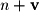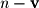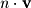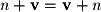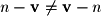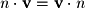Vector-Vector Binary Operations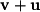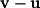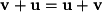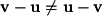Linear Combination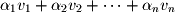Magnitude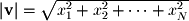Normalization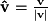Dot Product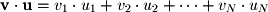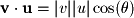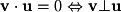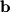-Component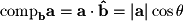Orthogonal Projection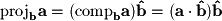You should know all of these definitions along with any other facts in the "important notes" column. I use the word "know" rather than "memorize" because most of these definitions can become intuitive if you take the time to understand what they mean and how they are derived. Then, once you understand them, formulas such as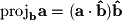are easy to put together in a few seconds in your head from the basic principles involved. On the other hand, if you do not learn these definitions and the ones in subsequent chapters in this way, you will find that linear algebra simply has too many definitions to memorize and apply. If you do follow this method, this course will be much easier.